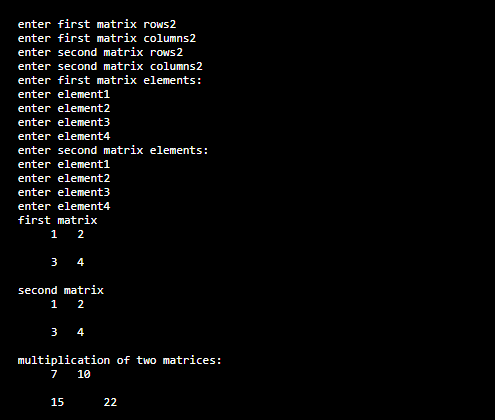# Python Matrix Multiplication

Here you will get program for python matrix multiplication.

If we want to multiple two matrices then it should satisfy one condition. We need to check this condition while implementing code without ignoring.

Amxn x Bpxq then n should be equal to p. Then only we can multiply matrices. Now we will see how to multiply two matrices using python nested list matrix representation.

## Python Matrix Multiplication

Below is python program to multiply two matrices.

Output### 8 thoughts on “Python Matrix Multiplication”

1.Senthil Selvan

Hi. I am new to python and i don’t understand why you have used end=” ” in this line
print(“\t”,matrix[i][j],end=” “)

also i tried the same prgm and without the end ” ” [because i got an error saying that end is not declared] and my o/p is always printed 1 element per line and not in matrix format. So what should i do ??

1.I have the same problem
but change the python format

1.python format???

2.to print in same line

2.URVASHI U Dhoot

This code does not check if 2 matrices can even be multiplied in the first place.
They can be multiplied only when No of Rows of 1st matirx == Columns of 2nd matrix

1.it does, as soon as m,n,p and q are taken as input

3.I don’t understand why we had to use ; while closing m , n, p, q.
Don’t we use ; only in CPP

4.why did we use ; to end m,n,p,q.